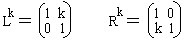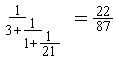# Continued Fractionson the Stern-Brocot Tree

The "left-side" and "right-side" encodings are complementary in more than one way. First of all, where one has letter L, the other has letter R, and vice versa. Secondly (and this explains left/right-side terminology), one applies to the tree (matrix) t on the left while the other applies on the right. There is a third difference between the two encodings that has to do with their utility. As we saw, the left-side encoding is useful in determining location of a fraction on the Stern-Brocot tree. The right-side encoding, on the other hand, leads directly to the fraction that occupies that location. To see how this is done I shall have to introduce simple algebraic notations and invoke the notion of continued fractions. As we shall see shortly, the right-side encoding is naturally interpreted in terms of simple continued fractions.

But first let's simplify encoding strings by using exponential notations. Let's, as is customary, write Lk for the string of k L's. Similarly, Rk will denote the string RR...R that consists of k R's. Thus, for example, the string LLRLLRRRL is replaced with L2R1L2R3L1. Of course L1 = L and also R1 = R. When symbols L and R are looked at as matrices, power notations are consistent with the standard usage in matrix algebra.

With the new notations, Algorithm 1 is reduced to the old acquaintance, Euclid's Algorithm. Indeed, as multiplication of integers is repeated addition, so division is repeated subtraction. For example, f(LRLL) = f(L1R1L2) = 4/7. Compare this with an application of Euclid's algorithm:

7 = 1·4 + 3
4 = 1·3 + 1
3 = 3·1

The first two 1's match nicely with the first two exponents in L1R1L2, the last term is off by 1. Let's get another example. tL3R1L20 = [1/4,21/83] and therefore f(L3R1L20) = 22/87. On the other hand,

87 = 3·22 + 21
22 = 1·21 + 1
21 = 21·1

(If you want to verify the calculations, it helps to note thatwhich is easily proven by induction).

Again, Euclid's algorithm produces the correct exponents, except that the last one is off by 1. Why this is so? In the latter example, on the last stage, Algorithm 1 keeps subtracting 1 first from 21, then from 20, and so on until the result is equal to 1 which takes 20 steps. This is one less than the quotient 21/1.

Recollect now that Euclid's algorithm also yields representation of continued fractions so that 22/87 = [3,1,21] which stands forTo sum up, a pure reformulation of the Algorithm 1 tells us that

(1)

f(La1Ra2... Lak) = [a1, a2, ..., ak+1]

Encodings of fractions on the right side of the tree (these all are greater than 1) start with R. According to our convention, we then use a semicolon to separate the integer part:

(2)

f(Ra1La2... Lak) = [a1; a2, ..., ak+1]

Note that it does not matter whether the last symbol of encoding is L or R. The result remains valid in the latter case also.The connection between the Stern-Brocot encoding and continued fraction representation of the fractions on the tree has very nice consequences. For instance, fractions [a1, a2, ..., ak] in the same row of the tree have the same sum of coefficients: a1 + a2 + ... + ak. This sum is one more than the row number. 4/7 = [1, 1, 3] and is located in the fourth row (4 = 5 -1.) 22/87 = [3, 1, 21] and is located in the row #24 (24 = 25 - 1). The reason of course is that the row number is just the number of subtractions performed by Algorithm 1 before it terminates.

In the Stern-Brocot tree, every fraction (except for 1/0 and 0/1) has two children: the left offspring and the right offspring that are located just below the fraction, one a little to the left, the other a little to the right from their parent. Knowing the continued fraction representation of the parent, we can easily find those of its offsprings. To see this, observe that, for continued fractions, we have the following identity

(3)

[a1, a2, ..., ak+1] = [a1, a2, ..., ak, 1]

Every finite continued fraction has two representations. Assume we are given a fraction with the last coefficient greater than 1, [a1, a2, ..., ak], ak >1. Write it in two ways as above

[a1, a2, ..., ak] and [a1, a2, ..., ak -1, 1]

Add 1 to the last coefficient in both representations:

[a1, a2, ..., ak+1] and [a1, a2, ..., ak -1, 2]

These are the offsprings of [a1, a2, ..., ak]. For example, 4/7 = [1, 1, 3] = [1, 1, 2, 1] has two offsprings [1, 1, 4] = 5/9 and [1, 1, 2, 2] = 7/12.Computationally, every fraction on the Stern-Brocot tree has two parents. These are the fractions whose mediant equals the given one. We can find these also. One of the parents is located in the row above the given fraction, another is more distant. The representation of the former is simply obtained by subtracting 1 from the last coefficient of the fraction. The representation of the distant parent is obtained by simply omitting the last coefficient. For example, 7/12 = [1, 1, 2, 2] is the mediant of two fractions [1, 1, 2, 1] = [1, 1, 3] = 4/7 and [1, 1, 2] = 3/5 which is easily verifiable. 5/9 = [1, 1, 4] is the mediant of [1, 1, 3] = 4/7 and [1, 1] = 1/2.

The part concerning the distant parent bears some explanation. I refer to Theorem 1 from the page on Continued Fractions. Let there be a fraction p/q = [a1, a2, ..., ak] = [a1, a2, ..., ak-1, 1]. Using the notations employed in Theorem 1 with k replaced by k + 1 and ak+1 = 1 we can write

p/q = pk+1/qk+1 = (pk + pk-1)/(qk + qk-1),

where pk/qk = [a1, a2, ..., ak-1] and pk-1/qk-1 = [a1, a2, ..., ak-1]. Plainly, this is just what we were looking for.### References

1. R. Graham, D. Knuth, O. Patashnik, Concrete Mathematics, 2nd edition, Addison-Wesley, 1994.
2. M. Schroeder, Fractals, Chaos, Power Laws, W.H.Freeman and Company, 1991